# Relative Velocity Formula

• Last Updated : 11 Mar, 2022

Let us suppose we are travelling on a Bus, let’s say, another bus overtakes us. We will not feel the actual speed of the overtaking bus, as felt by a person who looks it, standing by the side of the road. If both the buses are moving at the same speed in the same direction, a person in one bus observes a person in the other bus at rest, even though both are in motion. If both buses are in the opposite direction, then the passengers observe a greater speed, greater than their individual speed. In all these situations, we measure the relative velocities. Thus,

The relative is the velocity of one body with respect to another body. It is measured as the rate of change of position of a body with respect to another body.

In Mathematics, the relative velocity is the vector difference of the velocities between the two bodies.

### What is Relative Velocity?

The velocity of a body with respect to the velocity of another body is called the relative velocity of the first body with respect to the second.

Relative velocity = Velocity of the first body – Velocity of the second body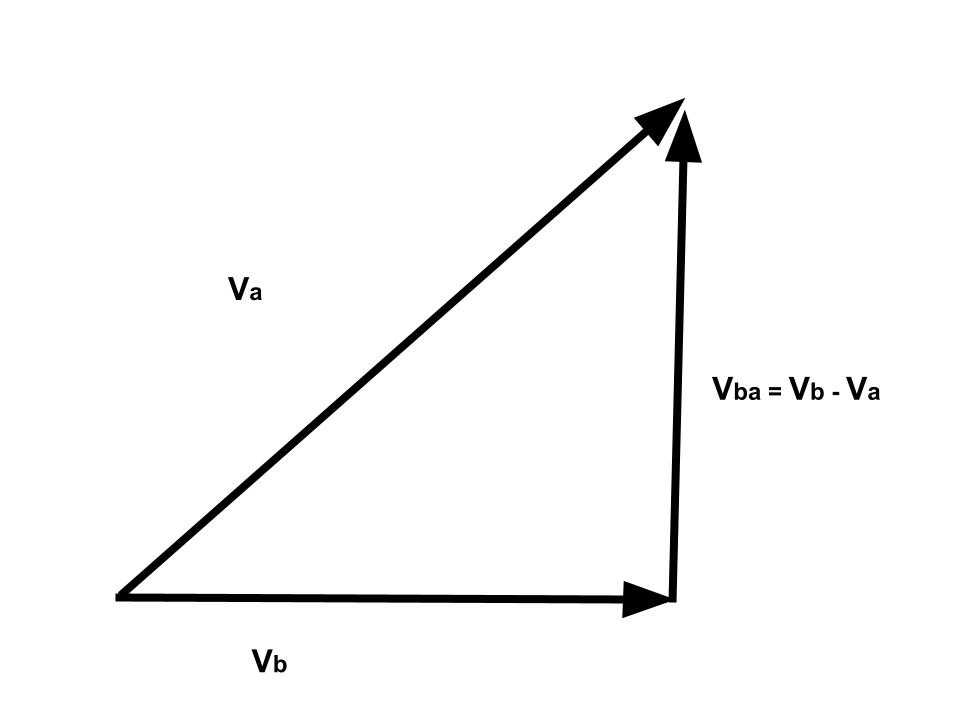If Va and Vb represent the velocities of two bodies A and B respectively at any instant, then, the relative velocity of A with respect to B is represented by Vab.

Then, Vab  = Va – Vb                                                                                                                                                                                               ……(1)

Similarly, the relative velocity of B with respect to A is given by,

Vba  = Vb – Va                                                                                                                                                                                                         ……(2)

So, from the equations (1) and (2), we can write

Vba  =  – Vab

This implies that

Relative velocity of A with respect to B = – Relative velocity of B with respect to A

Henceforth, the magnitude of both relative velocities are equal to each other.

• Difference between velocity and relative velocity: The difference between velocity and relative velocity is that velocity is measured with respect to a reference point which is relative to a different point. While relative velocity is measured in a frame where an object is either at rest or moving with respect to the absolute frame.
• Dimension Unit of relative velocity: The dimension Unit of relative velocity is similar to the unit of velocity may be expressed as – [ M0L1T-1].

Case 1: Relative Velocity for two objects moving in the same direction with equal velocities.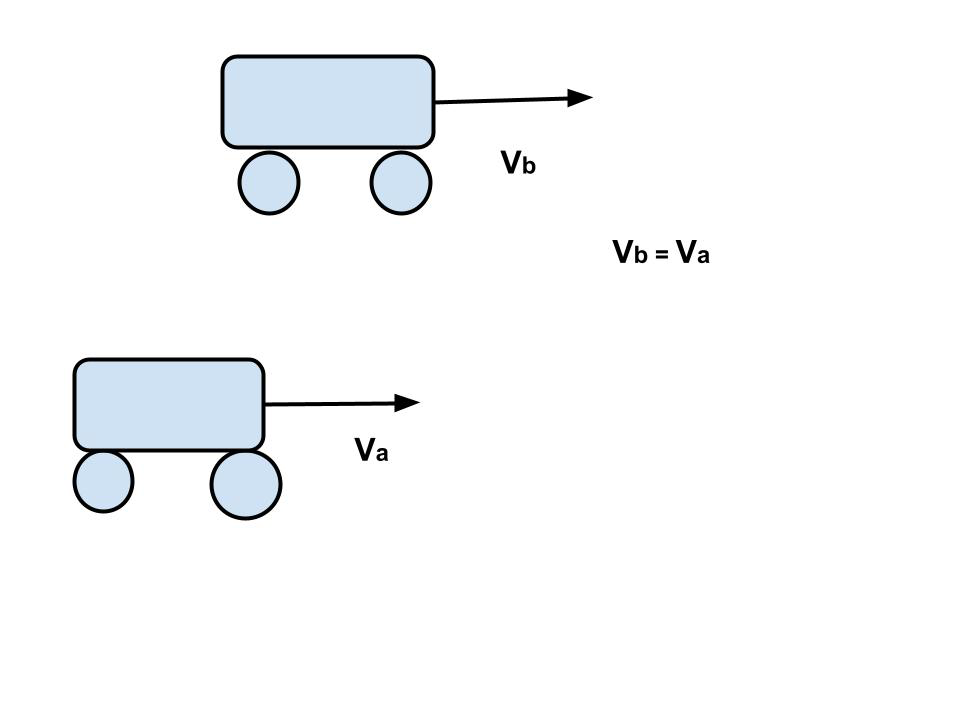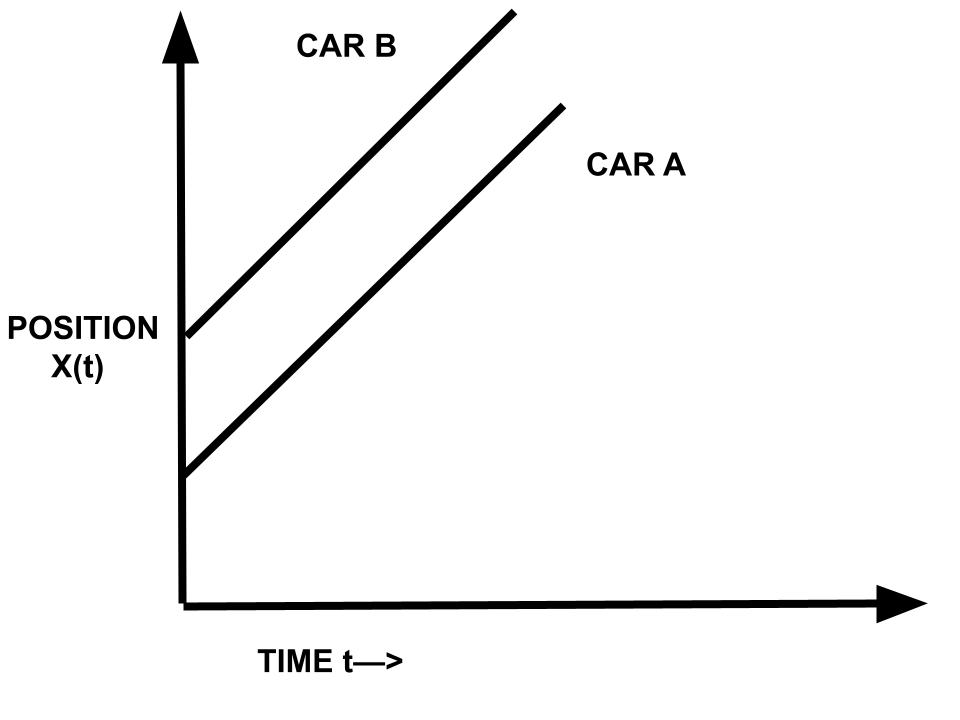Let’s now consider four different cases regarding relative motion. In the first case let us suppose two cars A and B are moving in the same direction with equal velocities (Va = Vb). For a person seated in A, the car B would appear to be at rest, if he forgets for a moment the fact that he himself is in motion. Hence the velocity of B relative to A is zero.

Vba= Vb– Va= 0 (as Va=Vb)

Similarly, in the case of a person seated in car B and observing car A. The relative velocity of A with respect to B is also zero.

Vab = Va– Vb = 0  (as Va=Vb)

So, if Vb – Va  = 0, means the two cars are moving in the same direction and are keeping the same distance between them always. So their position-time graphs are two parallel straight lines.

Case 2: Relative velocity for two objects starting from the same point and moving in the same direction with unequal velocities.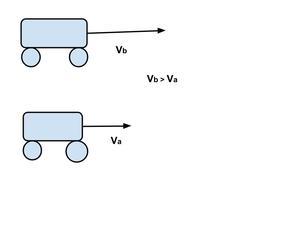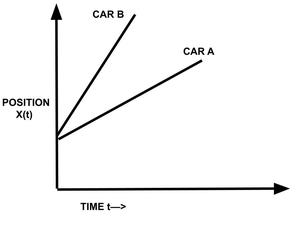In this case let Car A is moving with a velocity Va and Car B,(starting from the same point or X1(0)=X2(0) ), be moving with a greater velocity Vb in the same direction. Then, the person in car A feels that bus B is moving away from him with velocity,

Vba = Vb – Va

And for an observer in car B, car A appears to go back with a velocity,

Vab = Va-Vb = – (Vb– Va )

Velocity of A with respect to B= – (Velocity of B with respect to A)

That is, the velocity of A relative to B is the negative of the velocity of B relative to A.

Case 3: Relative velocity for two objects starting from different positions and moving in the same direction with unequal velocities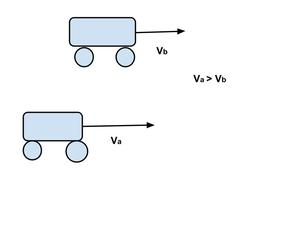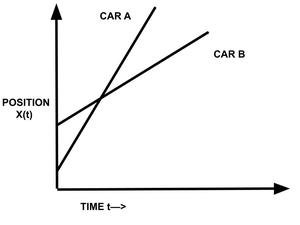Here, Va> Vb. If car A which is having the higher velocity and moving behind the car B, then car A will overtake the car B at some instant and the position-time graphs of the two cars will intersect at a point

Vab=Va-Vb ≠0 and

Vba=Vb-Va ≠0 as Va > Vb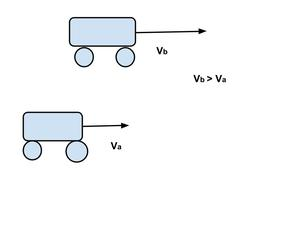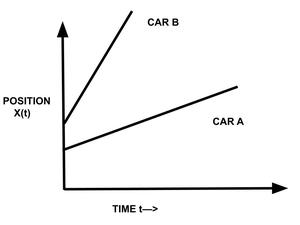And Vb > Va. If the car with greater velocity(in this case car B) is moving ahead, there is no chance of overtaking the other car A and hence the position-time graphs will not intersect, both the lines move far from each other as shown in the graph

Vab=Va-Vb ≠0 and

Vba=Vb-Va ≠0 as  Vb> Va

Clearly, If Vb – Va ≠ 0, means the two cars are moving with unequal velocities. Their position-time graphs are straight lines inclined to the time axis and one of them will be steeper than the other.

Case 4: Relative velocity for two objects moving in the opposite direction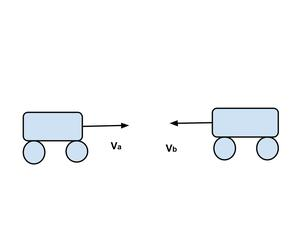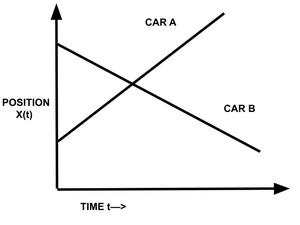If both the cars are moving in opposite directions as shown in the figure, the relative velocity of Vba or Vab will be

Vba=Vb-(-Va) = Vb+Va

Similarly,

Vab=Va-(-Vb) = Va+Vb

or

Vab=Vba=Va+Vb

It means, when two cars A and B are moving in opposite directions each seems to go very fast relative to the other.

### Sample Problems

Problem 1: Bus A travel with a speed of 40 m/s towards North and bus B travel with a speed of 60 m/s towards South. What is the relative velocity?

Solution:

Speed of bus A= Va = 40 m/s (towards north)

Speed of bus B= Vb = 60 m/s (towards south)

As both the buses are moving in opposite direction, so relative velocity is:

Vab = Va – (-Vb)

= 40 – (-60)

= 100 m/s

Problem 2: Two trucks, standing a few distances apart, start moving towards each other with speeds 1 m/s and 2 m/s along a straight road. What is the speed with which they approach each other?

Solution:

Let us consider that “A” denotes any truck at rest, “B” denotes first truck and “C” denotes second truck. The equation of relative velocity for this case is :

⇒ Vca = Vba + Vbc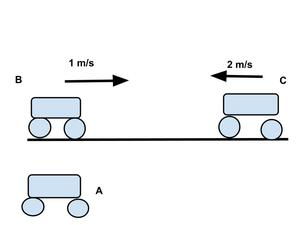Vba = Vb-Va =1-0=1m/s ,

Vca= Vba + Vbc=-2-0=-2m/s

Vba = 1 m/s and Vca= − 2 m/s

Using,

Vca  = Vba + Vcb

− 2 = 1 + Vcb

Vcb = − 2 − 1

= − 3 m/s

It means that the truck “C” is approaching “B” with a speed of -3 m/s along the straight road. Or, it means that the truck “B” is approaching “C” with a speed of 3 m/s along the straight road. We, therefore, say that the two trucks move towards each other each with a relative speed of 3 m/s.

Problem 3: Two cars, initially 900 m distant apart, start moving towards each other with speeds 1 m/s and 2 m/s along a straight road. When would they meet?

Solution:

The relative velocity of two cars (say 1 and 2) is:  V21 = V2-V1

Let us consider that the direction V1 is moving in a positive direction.

Here, V1 = 1 m/s and V2 = -2 m/s. So, relative velocity of two cars (of 2 w.r.t 1) is : ⇒ V21 = − 2 − 1 = − 3 m / s

This means that car “2” is approaching car “1” with a speed of -3 m/s along the straight road.

Similarly, car “1” is approaching car “2” with a speed of 3 m/s along the straight road. Therefore,

So can say that two cars are approaching at a speed of 3 m/s. Now, let the two cars meet after time:

t = Displacement ⁄ Relative velocity

= 900/3 = 30 sec.

Problem 4: How long will a girl sitting near the window of a train travelling at 36 km h-1 see a train passing by in the opposite direction with a speed of 18 km/h? The length of the slow-moving train is 90 m.

Solution:

Given : Velocity of train = Vt = 36 Km/h = 36 × 5/18 m/s = 10 m/s

Velocity of passenger moving in opposite direction = Vp = 18 Km/h = 18 × 5/18 m/s = 5 m/s

The relative velocity of the slow-moving train with respect to the girl is Vtp =  Vt – (-Vp) = Vt + Vp

Vtp =  (10 + 5) m/s = 15 m/s.

As the girl will watch the full length of the other train, to find the time taken to watch the full train:

Using relative speed = distance / time

15 = 90/t

t = 90/15

= 6 sec

Problem 5: A girl, moving at 3 km/hr along a straight line, finds that the raindrops are falling at 4 km/hr in the vertical direction. Find the angle with which raindrop hits the ground.

Solution:

Let the girl be moving in the x-direction. Let us also denote girl with “A” and raindrop with “B”. we need to know the direction of raindrop with respect to ground=Vb.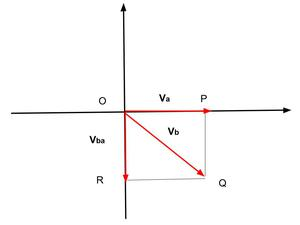Here Given

Va=3km/hr  , Vb =?  ,  Vba =4km/hr

Using equation, Vba = Vb-Va

So V=Va+Vba

In ΔOQR, Angle with which rain drop hits the ground = ∠QOR = θ

So tanθ= Va/Vba

tanθ=3/4

Hence,

θ= tan-1(3/4)

or

θ = 37°

Problem 6: A bus starting from a point travels towards the east with a velocity of 3 m/s. Another bus starting from the point travels towards the north with a  velocity. of 4 m/s. Find the magnitude of the relative velocity of one with respect to another.

Solution: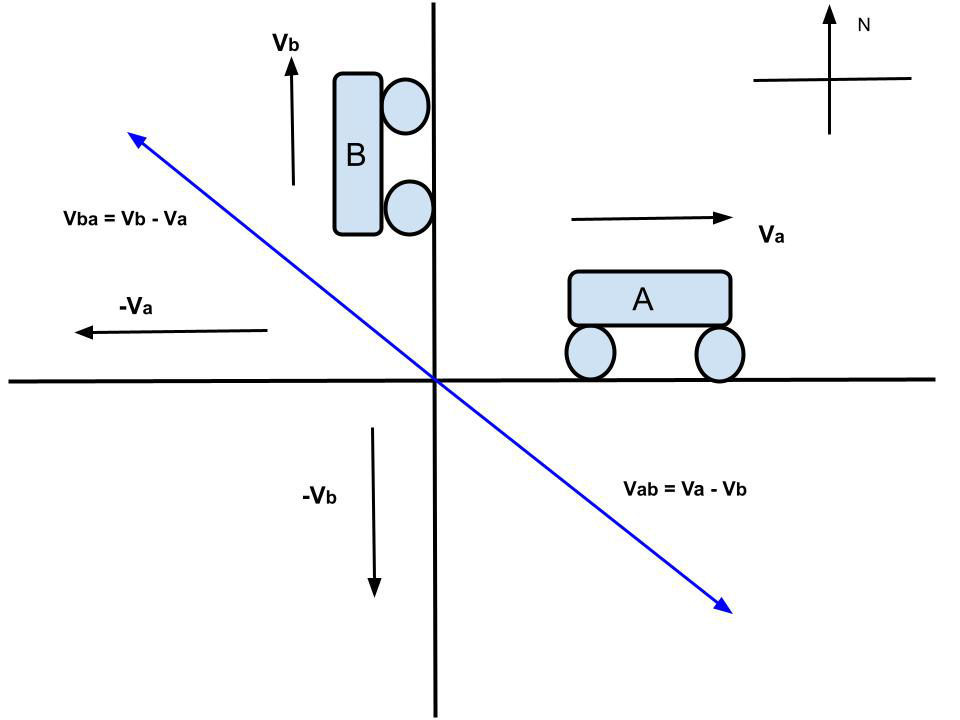Given that,

The Bus-A moves towards East with velocity = Va = 3 m/s

And Bus-B moves towards North with velocity = Vb =4 m/s

Now the relative velocity Vba of Bus-B with respect to Bus-A is towards the west of north direction as shown in the figure

Magnitude of Vba = √32+42  => Vba = 5 m/s

Similarly, relative velocity Vab of Bus-A with respect to Bus-B is towards the south of east direction as shown in the figure, so:

Magnitude of Vab = √42+3

Hence,

Vab = 5 m/s

My Personal Notes arrow_drop_up